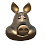### PlaneFit: Blender add-on to fit a plane through a set of vertices

After all the updates to my BlenderMarket add-ons, it is time to spend some time on other add-ons again.

## Fitting a plane

Fitting a plane through a collection of selected vertices might be useful in all sort of scenarios. Currently you can snap a plane to a face with Blender's snap tools but if we want to fit a plane to a large set of vertices, we need to resort to basic linear algebra to do this in a fast and robust manner. And because linear algebra is not everybody's favorite subject I created a small add-on.

After installation the add-on is available from the Add menu if you have a mesh in edit mode. Clicking on it will create a square plane that is fitted through all selected vertices. A size option lets you scale this new plane interactively. The result might look something like this:Note that currently we not check if the minimum of 3 vertices are selected, you get a an error if you try.

## Availability

As usual the add-on is available from my GitHub repository. (right-click on the link and select Save As .. to store the .py file somewhere where you can find it again )

## Source code

Both the linear algebra and the code to add a plane to an exiting mesh isn't completely trivial, so let me highlight the interesting bits:

### Fitting a plane

The fitting code is quite short and straight forward:
```import numpy as np

def planeFit(points):
ctr = points.mean(axis=0)
x = points - ctr
M = np.cov(x.T)
eigenvalues,eigenvectors = np.linalg.eig(M)
normal = eigenvectors[:,eigenvalues.argmin()]
return ctr,normal
```
Any book on linear algebra can give you a better explanation but with a fair bit of hand-waving it can be explained as follows: `points` is a list of 3d-vectors. `ctr` will be the midpoint of the plane which is the mean of each of the x, y and z-components.

In line 5 - 7 we calculate the eigen vectors of the point cloud. It is a 3d cloud so we will get 3 eigen vectors and 3 corresponding eigenvalues. Each combination of eigen value and eigen vector can be interpreted as a direction vector and a measure of how well it explains the spread of the points. This means that if the points lie roughly in a plane, the two biggest eigen vectors lie in the best fit plane while the smallest one will be the normal (all eigen vectors are perpendicular). And indeed this smallest one is the one we get in line 8.

### Adding a plane an existing mesh

Now adding a plane consisting of four vertices in a square would be quite simple, yes? Ehh, no: the `Mesh` object has a `from_pydata()` function but it only works correctly when adding to an initially empty mesh. So lines 15 - 27 essentially replicate what that function does: create 4 vertices, and 4 loops, compose a polygon out of it and add it to the mesh as well. We could have worked with a `BMesh` representation but then we would not have an efficient way to retrieve all vertex coordinates, something we really need when working with thousands of vertices.

The code in lines 7 - 12 is a very efficient way to get the coordinates of selected vertices into a Numpy array: we create Numpy arrays to hold all the vertex coordinates and the selected status, then get all of them with the fast built-in function `foreach_get()`. `verts[selected]` then leaves us with an array of just the coordinates of selected vertices, which we pass to our `planeFit()` function we saw earlier.

We then create two vectors perpendicular to our normal and use them to construct four vertex coordinates.

```         def execute(self, context):
bpy.ops.object.editmode_toggle()
me = context.active_object.data
count = len(me.vertices)
if count > 0:  # degenerate mesh, but better safe than sorry
shape = (count, 3)
verts = np.empty(count*3, dtype=np.float32)
selected = np.empty(count, dtype=np.bool)
me.vertices.foreach_get('co', verts)
me.vertices.foreach_get('select', selected)
verts.shape = shape
ctr, normal = planeFit(verts[selected])
dx, dy = orthopoints(normal)  # definition of orthopoints() not shown
# can't use mesh.from_pydata here because that won't let us ADD to a mesh
me.vertices[count  ].co = ctr+dx*self.size
me.vertices[count+1].co = ctr+dy*self.size
me.vertices[count+2].co = ctr-dx*self.size
me.vertices[count+3].co = ctr-dy*self.size
lcount = len(me.loops)
pcount = len(me.polygons)
me.polygons[pcount].loop_total = 4
me.polygons[pcount].loop_start = lcount
me.polygons[pcount].vertices = [count,count+1,count+2,count+3]
me.update(calc_edges=True)

bpy.ops.object.editmode_toggle()
return {'FINISHED'}```

1.Hello Michel,
Your script seems to be very usful for me,
But how can I make the script generate a new Mesh plane- not ADD it to the input mesh?
Is this possible as well?

1.no with this script that is not possible but because the vertices of the new plane are not connected to the rest of the mesh you could select 1 of the vertices, the do select connected (ctrl-L) followed by separate selected (that last one is in the select menu)

Hope this helps :-)

2.Hi Michel, thanks for the quick answer.
Yes, thats what I did so far (selecting a vert, Ctrl-L, seperate..) But this doesnt seem very comfortable...
Or to generate a new mesh for the plane from the start?
sadly I have close to no coding experience at all.. :(

1.I'll see what i can do. I am currently travelling so it might take some time though :-)

2.added feature as a 2nd menu entry. Current GitHub version is 201805050933

3.